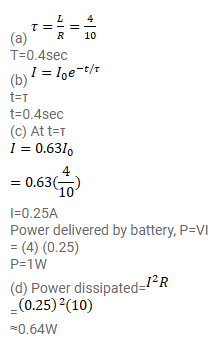# A solenoid having inductance

Question:

A solenoid having inductance $4.0 \mathrm{H}$ and resistance $10 \Omega$ is connected to a $4.0 \mathrm{~V}$ battery at $\mathrm{t}=0$. Find

(a) the time constant,

(b) the time elapsed before the current reaches $0.63$ of its steady-state value,

(c) the power delivered by the battery at this instant and

(d) the power dissipated in Joule heating at this instant.

Solution: